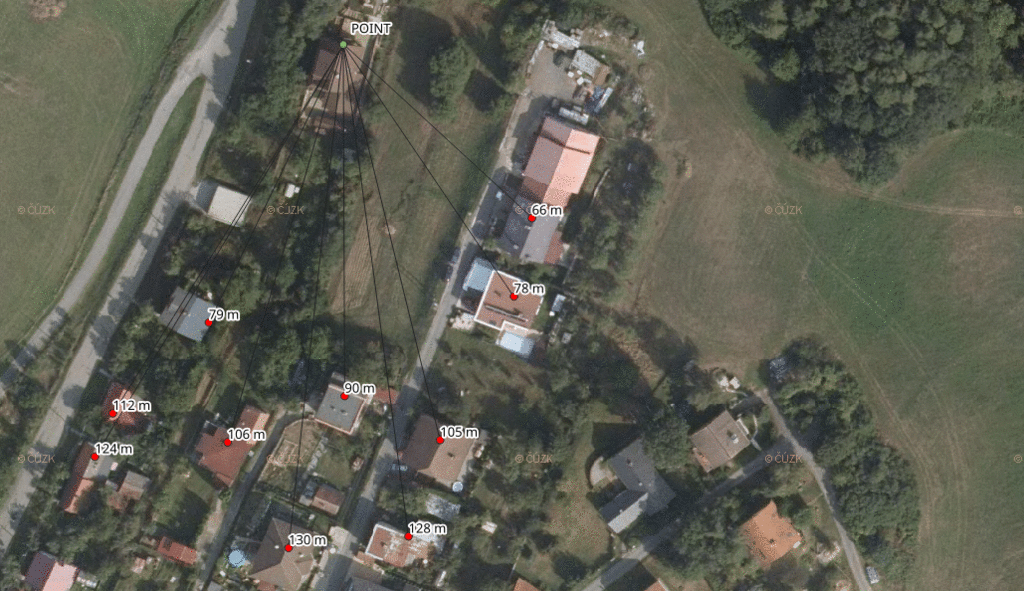# PostGIS Data Anonymization

Among all the sensitive spatial data being collected through cellphones and credit cards, our address of residency is probably the most delicate one. Can it be anonymized/pseudonymized/obscured before you share it with your business partners?

Imagine given a set of address points for each of your clients and the set of all address points in the country, you should adjust it in the following way:

• find the two nearest address points for each address point of your client
• find the center of these two and the client address point
• measure the distance of the computed center to each of three points and keep the maximum value
• make the biggest distance even bigger by adding 10 % of its value
• ceil the value
• output the new position and the ceiled distanceThis shifts each address point by a dynamic distance, giving us at least three points within the given distance (one of them being the original address point).

```SELECT
tmp.code,
ST_X(tmp.new_position) x,
ST_Y(tmp.new_position) y,
ceil(MAX(biggest_distance) + MAX(biggest_distance) * 0.1) round_distance
FROM (
SELECT
tmp.code,
tmp.geom,
ST_Centroid((ST_Union(two_closest_points, tmp.geom))) new_position,
-- get distance to two closest points and the client address point
ST_Centroid((ST_Union(two_closest_points, tmp.geom))) <-> (ST_DumpPoints(ST_Union(two_closest_points, tmp.geom))).geom biggest_distance
FROM (
SELECT
r1.code,
r1.geom,
ST_Union(neighbours.geom) two_closest_points
LATERAL (
-- keep two closest points to each client address point
SELECT
r2.code,
r2.geom,
r1.geom <-> r2.geom distance
WHERE r1.code <> r2.code
ORDER BY r1.geom <-> r2.geom ASC
LIMIT 2
) neighbours
GROUP BY
r1.code,
r1.geom
) tmp
) tmp
GROUP BY
tmp.code,
tmp.geom,
tmp.new_position;
```

You might want to use `LATERAL` for tasks like this.